# Regression Analysis

Regression analysis is a technique which investigates and models the relationship between a dependent variable (Y) to independent predictors (Xs). It can be used for hypothesis testing, modeling causal relationships (Y=f(x)) or prediction model. However, it is important to make sure that the underlying model assumptions are not violated. One of the key outputs in a regression analysis is the regression equation and correlation coefficients. The model parameters estimated from the data using the method of least squares. The model should also be checked for adequacy by reviewing the quality of the fit and checking residuals.

#### Explanation

To illustrate the concept of regression, let us use a simple model where a response, Y is linearly correlated to a single predictor, X. A straight line is drawn that best fits the data points.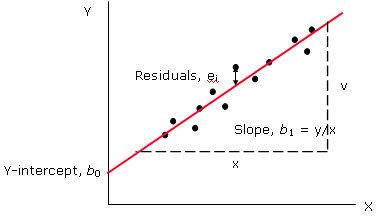In regression, the best-fit line is the straight line that minimises the sum of squared residuals. The fiitted model, Y = b0 + b1X will be estimated using the least square method, where b0 iis the intercept and b1 is the slope of the fitted line.

#### Assumptions

The following are the assumptions for regression analysis.

1. Predictors must be linearly independent (Any predictor cannot be expressed as a linear combination of the other predictors).
2. The residuals must be normally distributed, have constant variance and in control.
3. The sample must be random and representative of the population.

#### Models

The most common type of regression is simple linear regression that assumes a linear relationship between a single X and the Y. Multi-linear regression also gives a linear equation but includes several Xs. Non-linear regression is not commonly used except in instances where the relationship is driven by known physics of the relationship. Examples of different models are shown below:

• Simple Linear
• Linear: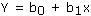• Quadratic: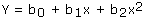• Cubic :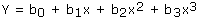• Multi-linear
• Multiple: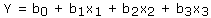• Involving interactions: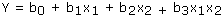• Non-linear Models
• Sine Waves: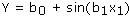• Exponential: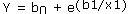#### How to do regression

The following are the guidelines to perform a regression analysis:

1. Visualise Correlation between Y and Xs
2. Selecting a model
3. Gather data on Y and Xs
4. Perform regression analysis
5. Optimize model
6. Accept or reject model

#### Data

The data on Y and Xs must be continuous. (Use logistic regression for non-continuous data) Collect data on Y. The range of data on Y and Xs collected should cover the entire operable range of investigation. This is because extrapolation is not advisable as the model derived may not be true beyond the data supplied to model the relationship.

#### Interpretation

There are several statistical software available to help you perform a regression analysis. The results of a regression analysis are rather standard and the following are guidelines on the results interpretation.

• SST - is the total sum of squares that represents the inherent variance within Y
• SSE - is the error sum of squares that represents the variance that cannot be explained by the model. A low value indicates a better model.
• SSR - is the regression sum of squares represents the variance that can be explained by the model. A high value indicates a better model.
• R-sq - is the amount of variation of Y explained by the model. A high R-sq value generally indicates (not guarantee) a good model.
• R-sq (adj) - R-sq may increase when a factor is added to the model, even if the factor is insignificant. R2(adj) adds a penalty for keeping insignificant factors in the model. Therefore a high R-sq (adj) value indicates a good model with less number of Xs.
• F - is the regression mean sum of squares and error mean sum of square ratio. A high value indicates a good model.
• P-value - is the probability value for corresponding F statistics. A low value indicates a good model.

#### Characteristics of a Good Model

A good model should have a high R2 value and the R2 (adj) should be fairly close. The residuals should be normally distributed with a mean of zero and a standard deviation of one. The data used for analysis should cover the entire range of response value for analysis and prediction. Finally, although the regression analysis is to find the best relationship between Y and Xs, it is also important to keep the model simple so that it can be meaningfully translated into the practical business world.

#### Improving the Model

The following are some of the possible ways to improve a model:

• Add More Predictors - One of the reasons for a poor model is the failure to identify and iinclude significant predictors in the analysis. This often requires a review of the physics of the process or experiment to identify the missing predictors that should have been included in the analysis. However, it is also important to bear in mind that by adding more predictors, the model may become more complex and less practical.
• Increase Power of Predictors - The model can also be improved by increasing the power of the predictors and using a quadratic or cubic model. However, this method should only be used if you understand the physics of the process you are trying to model. Otherwise, a quadratic or cubic model does not easily translate into the practical world.
• Remove Insignificant Predictors - Although removing insignificant and correlated predictors from the model does not increase the R 2 value, it does simply the model for practical application without a huge reduction in the R 2 value.

#### Caution

With the help of statistical software, performing regression analysis is relatively easy. However, it is always prudent to make sure that there is a correlation between Y and Xs (from correlation study or visualisation from scatter plot) and the relationship between Y and Xs makes sense, before performing a regression. The sample size used to estimate the model should also be sufficient. If data points are too few, a few outliers could dominate the analysis and lead to incorrect models being estimated. Finally, one of the most mistakes in regression which must be avoided is using the model to predict response value beyond the range of the data used in the analysis.

#### Demo Video

Multi-linear Regression